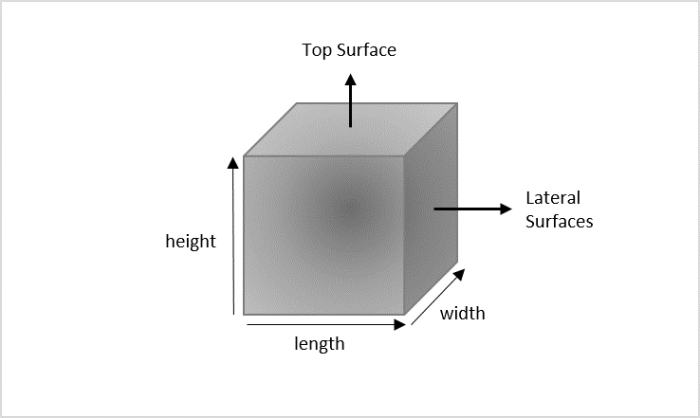# Python Program to calculate the area of Cube

To calculate the area of a cube, let us first revise the concept of a cube. A cube is a geometrical figure containing three dimensions: length, width, and height with all the dimensions having equal measurements. It has six square faces, four of which are lateral surfaces and the other two are the cube's top and bottom surfaces.The requirement to find the surface area is just knowing the length a single edge. The area of a cube is found using the following steps −

• Find the area of one square face with the given edge

• Four times the area of the square found is “lateral surface area

• Six times the area of the square found is “total surface area

Mathematical formulae to find surface areas of any cube with a given edge ‘a’ are as follows −

Lateral Surface Area: 4*(a)*(a)
Total Surface Area: 6*(a)*(a)


## Input Output Scenarios

Assume the length of the cube edge is positive, the output is obtained as −

Input: 6 // 6 is the edge length
Result: Lateral surface area: 144
Total surface area: 216


Assume the length of the cube edge is negative, the output is obtained as −

Input: -6 // -6 is the edge length
Result: Not a valid length


## Using Mathematical Formulae

To calculate the area of a Cube in Python using the mathematical formulae, we need to take the length of any edge in the cube as an input. Let us look at an example below −

### Example

In the python program given below, we are calculating both lateral and total surface areas of the Cube with a side length represented by “cube_edge”.

#The length of an edge in the Cube
cube_edge = 6

if(cube_edge > 0):

#Calculating Lateral Surface Area of the Cube
lsa = 4*(cube_edge)*(cube_edge)

#Calculating Total Surface Area of the Cube
tsa = 6*(cube_edge)*(cube_edge)

#Displaying the surface areas of the Cube
print("Lateral surface area: ", str(lsa))
print("Total surface area: ", str(tsa))
else:
print("Not a valid length")


### Output

On executing the python program above, the output is achieved as −

Lateral surface area: 144
Total surface area: 216


## Function to calculate Surface Area

In python, we can also make use of user-defined functions to display the surface areas of the cube. Functions in python are declared using the def keyword by passing single or multiple arguments separated by commas. Let us see how to find the surface area of the cube in the example below.

The syntax to declare a function is −

def function_name(argument 1, argument 2, …)


### Example

In the given example below, we declare two functions to calculate lateral and total surface areas of the cube. The length of a cube edge is passed as an argument to these functions.

#function to calculate lateral surface area
def lateral_surfacearea(cube_edge):
if(cube_edge > 0):
lsa = 4*(cube_edge)*(cube_edge)
print("Lateral surface area: ", str(lsa))
else:
print("Not a valid length")

#function to calculate total surface area
def total_surfacearea(cube_edge):
if(cube_edge > 0):
tsa = 6*(cube_edge)*(cube_edge)
print("Total surface area: ", str(tsa))
else:
print("Not a valid length")

lateral_surfacearea(4) #length of the edge = 4
total_surfacearea(4)


### Output

The output after calculating surface areas of the cube with edge length ‘4’ is displayed as −

Lateral surface area: 64
Total surface area: 96